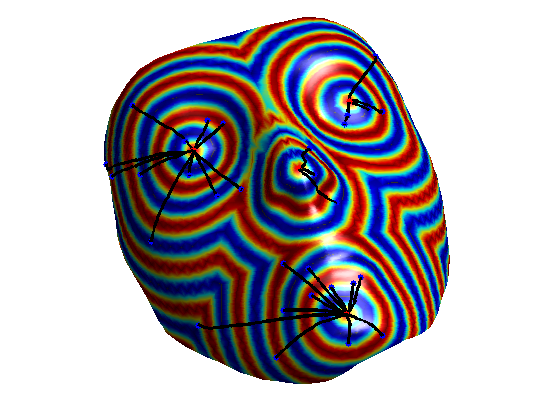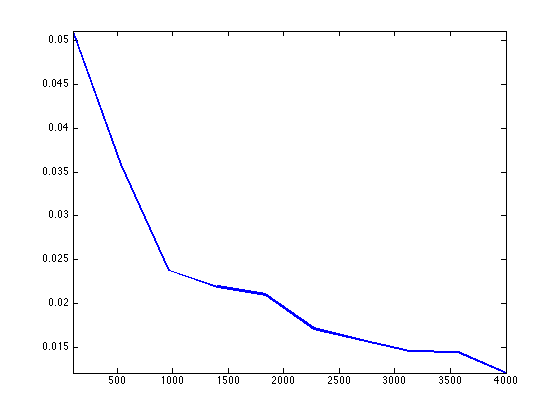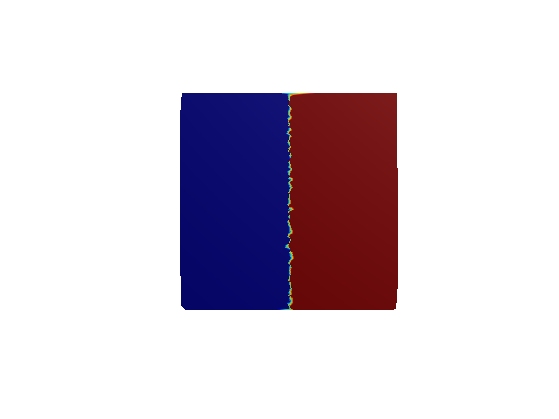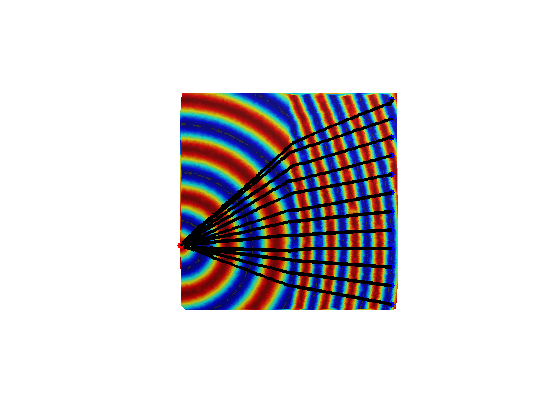$\newcommand{\NN}{\mathbb{N}} \newcommand{\CC}{\mathbb{C}} \newcommand{\GG}{\mathbb{G}} \newcommand{\LL}{\mathbb{L}} \newcommand{\PP}{\mathbb{P}} \newcommand{\QQ}{\mathbb{Q}} \newcommand{\RR}{\mathbb{R}} \newcommand{\VV}{\mathbb{V}} \newcommand{\ZZ}{\mathbb{Z}} \newcommand{\FF}{\mathbb{F}} \newcommand{\KK}{\mathbb{K}} \newcommand{\UU}{\mathbb{U}} \newcommand{\EE}{\mathbb{E}} \newcommand{\Aa}{\mathcal{A}} \newcommand{\Bb}{\mathcal{B}} \newcommand{\Cc}{\mathcal{C}} \newcommand{\Dd}{\mathcal{D}} \newcommand{\Ee}{\mathcal{E}} \newcommand{\Ff}{\mathcal{F}} \newcommand{\Gg}{\mathcal{G}} \newcommand{\Hh}{\mathcal{H}} \newcommand{\Ii}{\mathcal{I}} \newcommand{\Jj}{\mathcal{J}} \newcommand{\Kk}{\mathcal{K}} \newcommand{\Ll}{\mathcal{L}} \newcommand{\Mm}{\mathcal{M}} \newcommand{\Nn}{\mathcal{N}} \newcommand{\Oo}{\mathcal{O}} \newcommand{\Pp}{\mathcal{P}} \newcommand{\Qq}{\mathcal{Q}} \newcommand{\Rr}{\mathcal{R}} \newcommand{\Ss}{\mathcal{S}} \newcommand{\Tt}{\mathcal{T}} \newcommand{\Uu}{\mathcal{U}} \newcommand{\Vv}{\mathcal{V}} \newcommand{\Ww}{\mathcal{W}} \newcommand{\Xx}{\mathcal{X}} \newcommand{\Yy}{\mathcal{Y}} \newcommand{\Zz}{\mathcal{Z}} \newcommand{\al}{\alpha} \newcommand{\la}{\lambda} \newcommand{\ga}{\gamma} \newcommand{\Ga}{\Gamma} \newcommand{\La}{\Lambda} \newcommand{\Si}{\Sigma} \newcommand{\si}{\sigma} \newcommand{\be}{\beta} \newcommand{\de}{\delta} \newcommand{\De}{\Delta} \renewcommand{\phi}{\varphi} \renewcommand{\th}{\theta} \newcommand{\om}{\omega} \newcommand{\Om}{\Omega} \renewcommand{\epsilon}{\varepsilon} \newcommand{\Calpha}{\mathrm{C}^\al} \newcommand{\Cbeta}{\mathrm{C}^\be} \newcommand{\Cal}{\text{C}^\al} \newcommand{\Cdeux}{\text{C}^{2}} \newcommand{\Cun}{\text{C}^{1}} \newcommand{\Calt}{\text{C}^{#1}} \newcommand{\lun}{\ell^1} \newcommand{\ldeux}{\ell^2} \newcommand{\linf}{\ell^\infty} \newcommand{\ldeuxj}{{\ldeux_j}} \newcommand{\Lun}{\text{\upshape L}^1} \newcommand{\Ldeux}{\text{\upshape L}^2} \newcommand{\Lp}{\text{\upshape L}^p} \newcommand{\Lq}{\text{\upshape L}^q} \newcommand{\Linf}{\text{\upshape L}^\infty} \newcommand{\lzero}{\ell^0} \newcommand{\lp}{\ell^p} \renewcommand{\d}{\ins{d}} \newcommand{\Grad}{\text{Grad}} \newcommand{\grad}{\text{grad}} \renewcommand{\div}{\text{div}} \newcommand{\diag}{\text{diag}} \newcommand{\pd}{ \frac{ \partial #1}{\partial #2} } \newcommand{\pdd}{ \frac{ \partial^2 #1}{\partial #2^2} } \newcommand{\dotp}{\langle #1,\,#2\rangle} \newcommand{\norm}{|\!| #1 |\!|} \newcommand{\normi}{\norm{#1}_{\infty}} \newcommand{\normu}{\norm{#1}_{1}} \newcommand{\normz}{\norm{#1}_{0}} \newcommand{\abs}{\vert #1 \vert} \newcommand{\argmin}{\text{argmin}} \newcommand{\argmax}{\text{argmax}} \newcommand{\uargmin}{\underset{#1}{\argmin}\;} \newcommand{\uargmax}{\underset{#1}{\argmax}\;} \newcommand{\umin}{\underset{#1}{\min}\;} \newcommand{\umax}{\underset{#1}{\max}\;} \newcommand{\pa}{\left( #1 \right)} \newcommand{\choice}{ \left\{ \begin{array}{l} #1 \end{array} \right. } \newcommand{\enscond}{ \left\{ #1 \;:\; #2 \right\} } \newcommand{\qandq}{ \quad \text{and} \quad } \newcommand{\qqandqq}{ \qquad \text{and} \qquad } \newcommand{\qifq}{ \quad \text{if} \quad } \newcommand{\qqifqq}{ \qquad \text{if} \qquad } \newcommand{\qwhereq}{ \quad \text{where} \quad } \newcommand{\qqwhereqq}{ \qquad \text{where} \qquad } \newcommand{\qwithq}{ \quad \text{with} \quad } \newcommand{\qqwithqq}{ \qquad \text{with} \qquad } \newcommand{\qforq}{ \quad \text{for} \quad } \newcommand{\qqforqq}{ \qquad \text{for} \qquad } \newcommand{\qqsinceqq}{ \qquad \text{since} \qquad } \newcommand{\qsinceq}{ \quad \text{since} \quad } \newcommand{\qarrq}{\quad\Longrightarrow\quad} \newcommand{\qqarrqq}{\quad\Longrightarrow\quad} \newcommand{\qiffq}{\quad\Longleftrightarrow\quad} \newcommand{\qqiffqq}{\qquad\Longleftrightarrow\qquad} \newcommand{\qsubjq}{ \quad \text{subject to} \quad } \newcommand{\qqsubjqq}{ \qquad \text{subject to} \qquad }$

Geodesic Distance Computation on 3-D Meshes

# Geodesic Distance Computation on 3-D Meshes

This tour explores the computation of the geodesic distance on a mesh using an iterative algorithm to solve the Eikonal equation.

## Installing toolboxes and setting up the path.

You need to download the following files: signal toolbox, general toolbox, graph toolbox and wavelet_meshes toolbox.

You need to unzip these toolboxes in your working directory, so that you have toolbox_signal, toolbox_general, toolbox_graph and toolbox_wavelet_meshes in your directory.

For Scilab user: you must replace the Matlab comment '%' by its Scilab counterpart '//'.

Recommandation: You should create a text file named for instance numericaltour.sce (in Scilab) or numericaltour.m (in Matlab) to write all the Scilab/Matlab command you want to execute. Then, simply run exec('numericaltour.sce'); (in Scilab) or numericaltour; (in Matlab) to run the commands.

Execute this line only if you are using Matlab.

getd = @(p)path(p,path); % scilab users must *not* execute this


Then you can add the toolboxes to the path.

getd('toolbox_signal/');
getd('toolbox_general/');
getd('toolbox_graph/');
getd('toolbox_wavelet_meshes/');


## Triangulated Mesh

We consider a 3-D surface discretized using a triangular mesh with $$N$$ vertices. We denote $$\{x_i\}_{i=1}^{N}$$ the set of vertices, where $$x_i \in \RR^3$$. We denote $$\Ff \subset \{1, \ldots, N\}^3$$ the set of faces.

name = 'beetle'; % 1k
name = 'camel'; % 600 v
name = 'cow'; % 700v
name = 'venus'; % 700 v
name = 'mannequin'; % 400 v
name = 'nefertiti'; % 300 v
clear options; options.name = name;


Display.

clf;
options.lighting = 1;
plot_mesh(vertex0,faces0,options);Perform sub-division to obtained a finer mesh.

nsub = 2; % number of subdivision steps
options.sub_type = 'loop';
options.verb = 0;
[vertex,faces] = perform_mesh_subdivision(vertex0,faces0,nsub,options);
n = size(vertex,2);


Display.

clf;
options.lighting = 1;
plot_mesh(vertex,faces,options);Useful shortcuts.

dotp = @(u,v)sum(u.*v,1);
R = @(u)reshape(u, [1 1 length(u)]);


Shortcut for the explicit inverse of a series of 2D matrices, and the multiplication with a vector.

Inv1 = @(M,d)[M(2,2,:)./d -M(1,2,:)./d; -M(2,1,:)./d M(1,1,:)./d];
Inv  = @(M)Inv1(M, M(1,1,:).*M(2,2,:) - M(1,2,:).*M(2,1,:));
Mult = @(M,u)[M(1,1,:).*u(1,1,:) + M(1,2,:).*u(2,1,:);  M(2,1,:).*u(1,1,:) + M(2,2,:).*u(2,1,:)];


## Control Formulation of the Eikonal Equation

Our goal is to compute the geodesic distance to a set of starting points.

We consider an isotropic metric on the mesh $$W_i > 0$$.

Given a set $$I$$ of starting point, we want to compute the discrete geodesic distance map $$U \in \RR^N$$ to the starting points $$\{x_i\}_{i \in I}$$.

Let's consider a geodesic distance $$d(x,y)$$ on a manifold. A general mathematical result is that the geodesic distance $d(x,A) = \umin{a \in A} d(x,a)$ to a set $$A$$ is the unique solution to the following equation $\forall \, x \notin A, \quad d(x,A) = \umin{ y \in B(x) } d(y,A) + d(x,y)$ where $$B(x)$$ is a small disk around $$x$$ that does not contain $$A$$.

In a discrete setting, one can use this property with $$B(x_i)$$ being the one ring, made of the edges connecting vertices that are around $$x_i$$. Using a piecewise linear interpolation of $$U$$ on the mesh, one obtain that $$U$$ is a solution to a fixed point equation $U = \Ga(U)$ where the update operator $$\Ga : \RR^N \rightarrow \RR^N$$ is defined as $\Ga(U)_i = \umin{ (i,j,k) \in \Ff } d_{i,j,k}$ where $d_{i,j,k} = \umin{0 \leq t \leq 1} t U_{j} + (1-t) U_k + W_i \norm{ t x_j + (1-t) x_k - x }.$ The quantities $$d_{i,j,k}$$ can in fact be computed by solving a second order polynomial, as we describe in the following section.

## Geodesic distance Computation Using Jacobi Iterations

The mapping $$\Ga$$ is monotone $U \leq V \qarrq \Ga(U) \leq \Ga(V).$ If one uses an initialization $$U^{(0)}$$ such that $$\Ga(U^{(0)}) \geq U^{(0)}$$ (for instance setting $$U^{(0)}=0$$), then the iterates $U^{(\ell+1)} = \Ga(U^{(\ell)})$ are increasing. One can prove that they are bounded, and hence they converge to the (unique) fixed point solution of $$\Ga(U)=U$$.

Define the metric $$W_i > 0$$ on the mesh. We start with the uniform (constant) metric.

W = ones(n,1);


Indexes $$I \subset \{1,\ldots,N\}$$ of starting points. Warning: this list works for the mesh nefertitit refined twice. For other meshes, use the function select3dtool to retrive the indexes of a few vertices.

I = [88 2602 883 23];


Initialization of the distance map.

U = zeros(n,1);


Computation of the set of indexes for $$i,j,k$$.

i = [faces(1,:) faces(2,:) faces(3,:) ];
j = [faces(2,:) faces(3,:) faces(1,:) ];
k = [faces(3,:) faces(1,:) faces(2,:) ];


x stores the position of all the $$x_i$$ vertices (point where the value of $$d=d_{i,j,k}$$ is computed), while x1 and x2 store respectively $$x_j-x_i$$ and $$x_k-x_i$$.

x  = vertex(:,i);
x1 = vertex(:,j) - x;
x2 = vertex(:,k) - x;


ui stores $$U_{j}$$, uk stores $$U_k$$, w stores $$w=W_i$$. We denote $$u=(U_j,U_k) \in \RR^2$$.

uj = U(j);
uk = U(k);
u = [R(uj); R(uk)];
w = R( W(i) );


C stores the inner product matrix $$C_{s,t} = \dotp{x_s}{x_t}$$, and we denote $$S = C^{-1}$$.

C = [R(dotp(x1,x1)) R(dotp(x1,x2)); R(dotp(x2,x1)) R(dotp(x2,x2))];
S = Inv(C);


If we denote $$g = \al_1 x_1 + \al_2 x_2$$ the (unknown) update direction, the geodesic distance (which is affine in the triangle), is written as $$U(z) = \dotp{g}{z-x} + d$$ where $$d \in \RR$$ is unknown.

We need to compute both $$g$$ and $$d$$. Since $$U(x_j)=u_1$$ and $$U(x_k)=u_2$$, one obtains $\al = S ( u - d \mathbb{I} ),$ where $$\mathbb{I} = (1,1) \in \RR^2$$. Making use of the Eikonal equation $$\norm{\nabla U(x)} = \norm{g} = w$$, one obtains that $$d$$ is the (maximum) solution of the following second order equation $a d^2 - 2 b d + c = 0 \qwhereq \choice{ a = \dotp{S \mathbb{I}}{\mathbb{I}} \\ b = \dotp{S \mathbb{I}}{u} \\ c = \dotp{S u}{u} - w^2 }$

Compute the values of $$a, b, c$$.

a = sum(sum(S));
b = dotp( sum(S,2), u );
c = dotp( Mult(S,u), u ) - w.^2;


Compute the reduced discriminant $$\de = b^2 - a c$$, which is always positive.

delta = max( b.^2 - a.*c, 0);


The solution is then $d = \frac{ b + \sqrt{\delta} }{ a }.$

d = (b + sqrt(delta) )./a;


To ensure correctness of the scheme, the update should come from within the triangle (which correspond to the condition $t \in [0,1]$ in the original definition of $$d_{i,j,k}$$ using the control formulation). This corresponds to having $$\al_1<=0$$ and $$\al_2 \leq 0$$ where $$\al = S ( u - d \mathbb{I} )$$.

Compute $$\al \in \RR^2$$.

alpha = Mult( S, u - repmat(d, 2, 1) );


For the index $$i \in J$$ where the update comes from outside the edge $$[x_j,x_k]$$, we use an update along the edge $d = \min\pa{ U_j + \norm{x-x_j}W_i, U_k + \norm{x-x_k}W_i }.$

J = find( alpha(1,1,:)>0 | alpha(2,1,:)>0 );
d1 = sqrt(dotp(x1,x1)); d1 = d1(:).*w(:) + uj(:);
d2 = sqrt(dotp(x2,x2)); d2 = d2(:).*w(:) + uk(:);
d = d(:);
d(J) = min(d1(J), d2(J));


Now that d stores the value of d_{i,j,k} for a bunch of faces assign in U1(i) the mininimum between the previous U1(i) and all the entries in d that corresponds to a face $$(i,j,k)$$.

U1 = accumarray(i', d, [n 1], @min);  U1(U1==0) = Inf;


Enforce the boundary conditions $$\forall i \in I, \, U_i = 0$$.

U1(I) = 0;


Update the solution.

U = U1;


Exercice 1: (check the solution) Perform the full iterative algorithm until convergence. Store in err(l) the fixed point error $$\norm{ U^{(\ell+1)} - U^{(\ell)} }$$. Note: you might want to put outside of the loop all the quantities that do not depend on $$u$$, e.g. $$S, a,$$ etc.

exo1;Display the fixed point energy.

clf;
h = plot(err);
set(h, 'LineWidth', 2);
axis tight;For the display of the distance.

mycolor = @(U,k)mod(U/max(U), 1/k);
mycolor = @(U,k)cos(2*pi*k*U/max(U));


Display the distance map.

clf;
options.face_vertex_color = mycolor(U, 5);
plot_mesh(vertex,faces,options);
colormap jet(256);## Geodesic Path Extraction

A geodesic minimal path from any point on the mesh to the starting point can be computed using a gradient descent. Since the computed geodesic distance is affine on each triangle, it makes sense to define a discretized path that is a line segment on each face.

Extract minimal paths from all over the mesh, starting from the boundary.

k = 30;
pend = round(rand(k,1)*n)+1;
options.method = 'continuous';
paths = compute_geodesic_mesh(U, vertex, faces, pend, options);


Display.

clf;
plot_fast_marching_mesh(vertex,faces, mycolor(U, 5), paths, options);## Geodesic Distance Convergence

The discrete geodesic distance converges to the continuous distance when the mesh is refined.

Compute a Delaunay triangulation of random point on a square with the first point in the center.

n = 3000;
vertex = [2*rand(2,n)-1; zeros(1,n)];
vertex(:,1) = 0;
faces = compute_delaunay(vertex);
options.name = '';
I = 1;


Display.

clf
plot_mesh(vertex,faces);Exercice 2: (check the solution) Compute and display the geodesic distance.

exo2;Exercice 3: (check the solution) Display the convergence of the computed geodesic distance to the the true geodesic distance (which is the Euclidean distance $$\norm{x_i}$$) as $$n$$ increases. Note: the triangulation with increasing number of points should be refining (i.e. a finer triangulation should contains all the other ones).

exo3;## Spacially Varying Metrics

One can define a non-constant metric on the mesh.

Triangulation of a square.

n = 8000;
vertex = [rand(2,n); zeros(1,n)];
vertex(:,1) = [0 .3 0]';
faces = compute_delaunay(vertex);
I = 1;


Generate a binary metric in order to produce a reflexion effect.

W = ones(n,1);
W(vertex(1,:)<.5) = 1/2;


Display the metric.

options.face_vertex_color = W;
clf;
plot_mesh(vertex,faces,options);
colormap jet(256);Exercice 4: (check the solution) Compute the geodesic distance for a metric $$W_i$$ that is not constant over the mesh.

exo4;